## Now to create scatter plot like this?

HI, dose anyone has insight to create the following figure?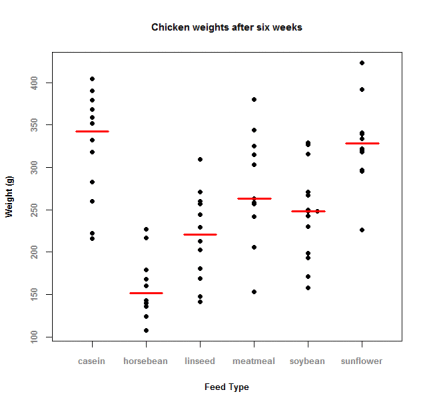1 ACCEPTED SOLUTION

Accepted SolutionsPGStats
Opal | Level 21

## Re: Now to create scatter plot like this?

OK, one more try, following @Jay54's suggestion (tested with 9.4) :

``````proc sql;
create table class as
select *, median(weight) as med
from sashelp.class
group by age;
quit;

proc sgplot data=class noautolegend;
highlow x=age high=med low=med / type=bar lineattrs=(thickness=2 color=red) barwidth=0.6;
scatter y=weight x=age / markerattrs=(symbol=circlefilled color=blue);
xaxis type=discrete;
run;
``````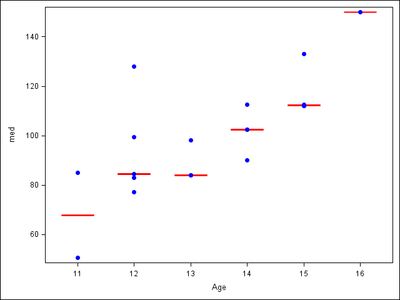PG
11 REPLIES 11

## Re: Now to create scatter plot like this?

We could try the sgplot procedure as below,  I am assuming that the graph is overlapping of scatter plot on box plot with mean. If it is the case the below procedure should help.

proc sgplot data=have;

scatter x=feed_type y=weight /  markerattrs = (size = 2 symbol = circlefilled); /*this will be the scatter plot*/

vbox weight / category=feed_type; /*this will create the box plot with mean*/

quit;

run;

Thanks,
Jag

## Re: Now to create scatter plot like this?

It seems that the overlapping scatter plot and box plot is not worked.
Please see the SAS log below.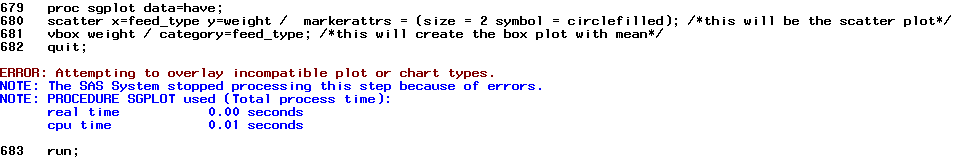By the way, I'm using SAS 9.3.PGStats
Opal | Level 21

## Re: Now to create scatter plot like this?

I guess in 9.3 you could cheat this way:

``````proc sql;
create table class as
select *, median(weight) as med
from sashelp.class
group by age;
quit;

proc sgplot data=class noautolegend;
scatter x=age y=med / yerrorlower=med yerrorupper=med
markerattrs=(size=0) errorbarattrs=(thickness=2 color=red);
scatter y=weight x=age / markerattrs=(symbol=circlefilled color=blue);
run;``````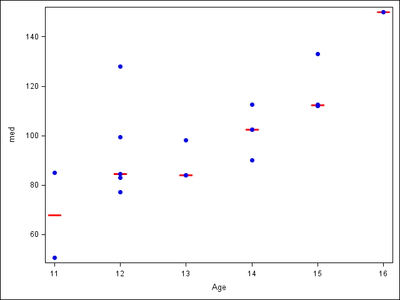PG

## Re: Now to create scatter plot like this?

Hi PG Stats,

Another question, how to lengthen the length of 'mean'?PGStats
Opal | Level 21

## Re: Now to create scatter plot like this?

I don't know. Seems to be proportional to line thickness.

PG

## Re: Now to create scatter plot like this?

Okay. I will try.
According to your reply, SAS 9.3 cannot overlay vbox and scatter plot, right?

## Re: Now to create scatter plot like this?PGStats
Opal | Level 21

## Re: Now to create scatter plot like this?

Here is an approaching example:

``````proc sql;
create table class as
select *, median(weight) as med
from sashelp.class
group by age;
quit;

proc sgplot data=class noautolegend;
vbox med / category=age nomean nomedian nocaps
lineattrs=(color=red thickness=2);
scatter y=weight x=age / markerattrs=(symbol=circlefilled);
xaxis type=discrete;
run;``````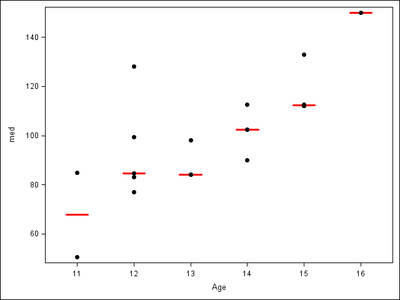PGJay54
Meteorite | Level 14

## Re: Now to create scatter plot like this?

If you know the value for drawing the mean or median (the red line), and are using SAS 9.3, I suggest overlaying a Scatter and a HighLow (Type=Bar).  Make the high and low the same value to get a single line.  You can set the BarWidth to control the width of the line.PGStats
Opal | Level 21

## Re: Now to create scatter plot like this?

OK, one more try, following @Jay54's suggestion (tested with 9.4) :

``````proc sql;
create table class as
select *, median(weight) as med
from sashelp.class
group by age;
quit;

proc sgplot data=class noautolegend;
highlow x=age high=med low=med / type=bar lineattrs=(thickness=2 color=red) barwidth=0.6;
scatter y=weight x=age / markerattrs=(symbol=circlefilled color=blue);
xaxis type=discrete;
run;
``````PG

## Re: Now to create scatter plot like this?

I'm creating the figure by your suggestion. Great thanks for everyone's suggestion!
Besides, the function "median" seems not okay in SAS 9.3. There will be a warning message "The MEDIAN function has been called with only one argument.".
However, 'mean' function can be worked in proc sql statement and that what I need!

Thanks again!

JC
Discussion stats
• 11 replies
• 4994 views
• 5 likes
• 5 in conversation